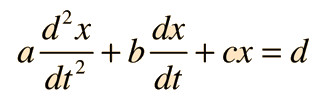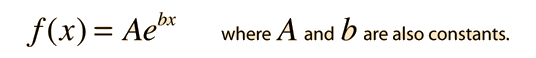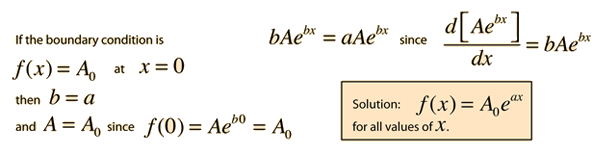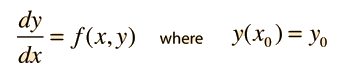# Differential Equations

A differential equation is an equation which contains the derivatives of a variable, such as the equationHere x is the variable and the derivatives are with respect to a second variable t. The letters a, b, c and d are taken to be constants here. This equation would be described as a second order, linear differential equation with constant coefficients. It is second order because of the highest order derivative present, linear because none of the derivatives are raised to a power, and the multipliers of the derivatives are constant. If x were the position of an object and t the time, then the first derivative is the velocity, the second the acceleration, and this would be an equation describing the motion of the object. As shown, this is also said to be a non-homogeneous equation, and in solving physical problems, one must also consider the homogeneous equation.

Index

 HyperPhysics****HyperMath*****Differential equations R Nave
Go Back

# First Order Homogeneous DE

A first order homogeneous differential equation involves only the first derivative of a function and the function itself, with constants only as multipliers. The equation is of the formand can be solved by the substitutionThe solution which fits a specific physical situation is obtained by substituting the solution into the equation and evaluating the various constants by forcing the solution to fit the physical boundary conditions of the problem at hand. Substituting gives### Applications

 First order non-homogeneous equation
Index

 HyperPhysics****HyperMath*****Differential equations R Nave
Go Back

# General Solution to a D.E.

The general solution to a differential equation must satisfy both the homogeneous and non-homogeneous equations. It is the nature of the homogeneous solution that the equation gives a zero value. If you find a particular solution to the non-homogeneous equation, you can add the homogeneous solution to that solution and it will still be a solution since its net result will be to add zero. This does not mean that the homogeneous solution adds no meaning to the picture; the homogeneous part of the solution for a physical situation helps in the understanding of the physical system. A solution can be formed as the sum of the homogeneous and non-homogeneous solutions, and it will have a number of arbitrary (undetermined) constants. Such a solution is called the general solution to the differential equation. For application to a physical problem, the constants must be determined by forcing the solution to fit physical boundary conditions. Once a general solution is formed and then forced to fit the physical boundary conditions, one can be confident that it is the unique solution to the problem, as gauranteed by the uniqueness theorem.
Index

 HyperPhysics****HyperMath*****Differential equations R Nave
Go Back

# Boundary Conditions

The boundary conditions on a differential equation are the constraining values of the function at some particular value of the independent variable. For example, if the equation involves the velocity, the boundary condition might be the initial velocity, the velocity at time t=0. In order to have a complete solution, there must be a boundary condition for each order of the equation - two boundary conditions for a second order equation, only one necessary for a first order differential equation. If a solution to a differential equation is found which satisfies all the boundary conditions, then it is the only solution to that equation - this is called the uniqueness theorem. Therefore, a reasonable approach to finding solutions to differential equations in physical problems is to use a trial solution and try to force it to fit the boundary conditions. If successful, then this approach finds the unique solution.
Index

 HyperPhysics****HyperMath*****Differential equations R Nave
Go Back

# Uniqueness Theorem

For the differential equations applicable to physical problems, it is often possible to start with a general form and force that form to fit the physical boundary conditions of the problem. This kind of approach is made possible by the fact that there is one and only one solution to the differential equation, i.e., the solution is unique.

Stated in terms of a first order differential equation, if the problemmeets the condition such that f(x,y) and the derivative of y is continuous in a given rectangle of (x,y) values, then there is one and only one solution to the equation which will meet the boundary conditions.

Index

 HyperPhysics****HyperMath*****Differential equations R Nave
Go Back

# Differential Equation Terminology

Some general terms used in the discussion of differential equations:

Order: The order of a differential equation is the highest power of derivative which occurs in the equation, e.g., Newton's second law produces a 2nd order differential equation because the acceleration is the second derivative of the position.

Linear and nonlinear: A differential equation is said to be linear if each term in the equation has only one order of derivative, e.g., no term has both y and the derivative of y with respect to time. Also, no derivative is raised to a power.

Homogeneous and nonhomogeneous: A differential equation is said to be homogeneous if there is no isolated constant term in the equation, e.g., each term in a differential equation for y has y or some derivative of y in each term.

Index

 HyperPhysics****HyperMath*****Differential equations R Nave
Go Back